# Chapter 11 Facets

One of the most useful techniques in data visualization is rendering groups of data alongside each other, making it easy to compare the groups. With ggplot2, one way to do this is by mapping a discrete variable to an aesthetic, like x `position`, `color`, or `shape.` Another way of doing this is to create a subplot for each group and draw the subplots side by side.

These kinds of plots are known as Trellis displays. They’re implemented in the lattice package as well as in the ggplot2 package. In ggplot2, they’re called facets. In this chapter I’ll explain how to use them.

## 11.1 Splitting Data into Subplots with Facets

### 11.1.1 Problem

You want to plot subsets of your data in separate panels.

### 11.1.2 Solution

Use `facet_grid()` or `facet_wrap()`, and specify the variables on which to split.

With `facet_grid()`, you can specify a variable to split the data into vertical subpanels, and another variable to split it into horizontal subpanels (Figure 11.1):

``````# Create the base plot
mpg_plot <- ggplot(mpg, aes(x = displ, y = hwy)) +
geom_point()

# Faceted by drv, in vertically arranged subpanels
mpg_plot +
facet_grid(drv ~ .)

# Faceted by cyl, in horizontally arranged subpanels
mpg_plot +
facet_grid(. ~ cyl)

# Split by drv (vertical) and cyl (horizontal)
mpg_plot +
facet_grid(drv ~ cyl)``````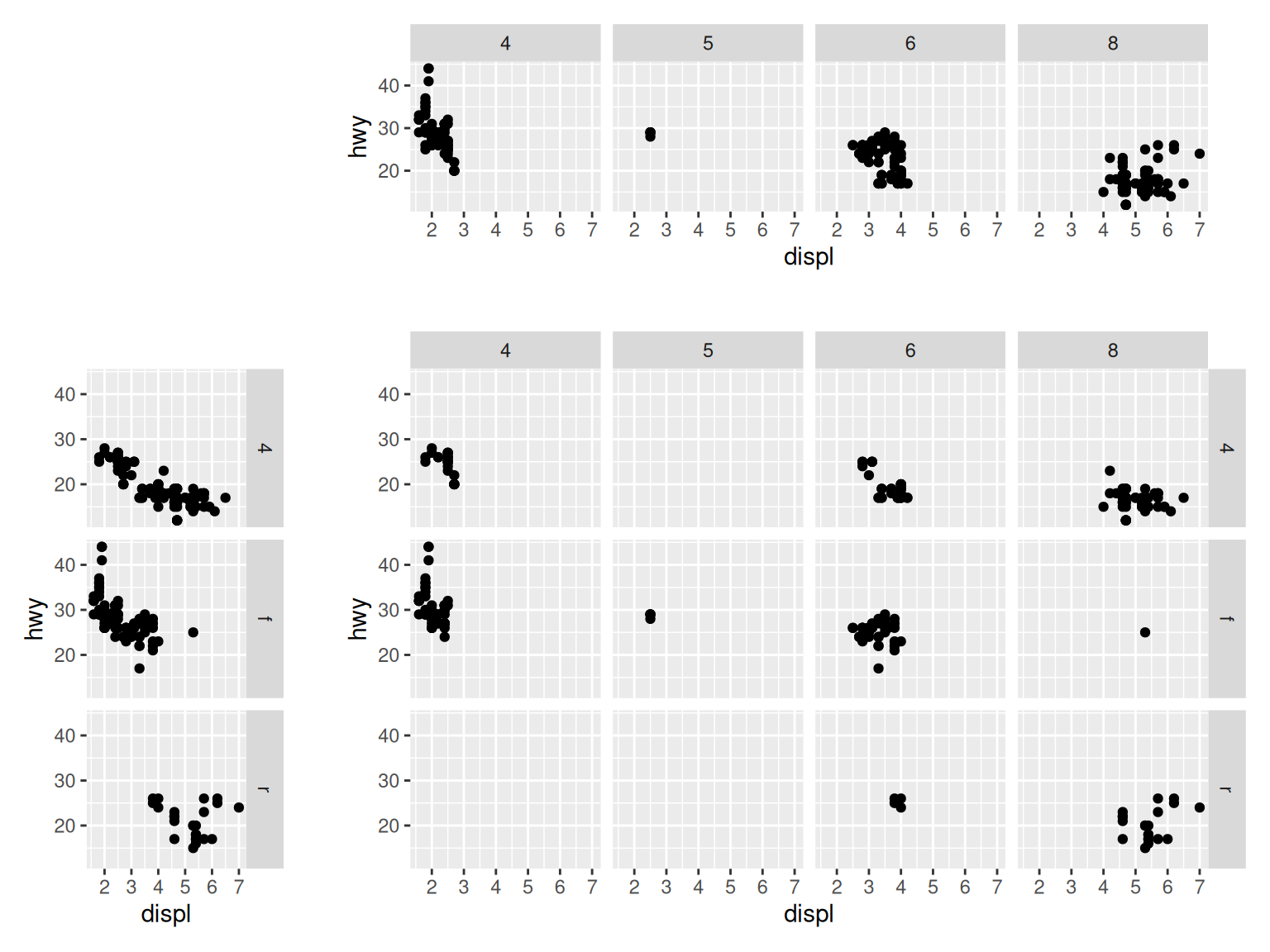Figure 11.1: Faceting horizontally by drv (top); Faceting vertically by cyl (middle); Faceting in both directions, with both variables (bottom)

With `facet_wrap()`, the subplots are laid out horizontally and wrap around, like words on a page, as in Figure 11.2:

``````# Facet on class
# Note that there is nothing before the tilde
mpg_plot +
facet_wrap( ~ class)``````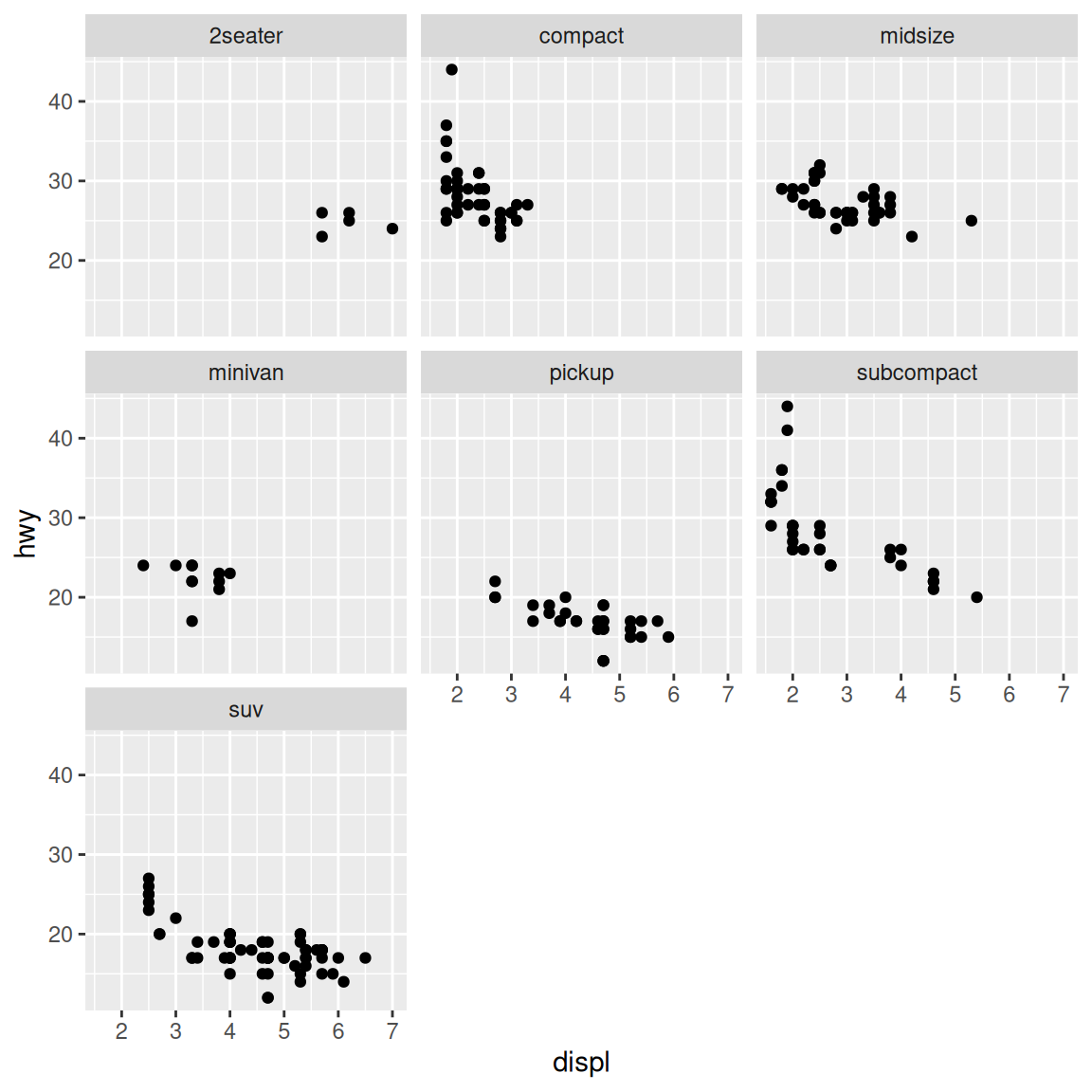Figure 11.2: A scatter plot with `facet_wrap()` on class

### 11.1.3 Discussion

With `facet_wrap()`, the default is to use the same number of rows and columns. In Figure 11.2, there were seven facets, and they fit into a 3×3 square. To change this, you can pass a value for `nrow` or `ncol`:

``````# These will have the same result: 2 rows and 4 cols
mpg_plot +
facet_wrap( ~ class, nrow = 2)

mpg_plot +
facet_wrap( ~ class, ncol = 4)``````

The choice of faceting direction depends on the kind of comparison you would like to encourage. For example, if you want to compare heights of bars, it’s useful to make the facets go horizontally. If, on the other hand, you want to compare the horizontal distribution of histograms, it makes sense to make the facets go vertically.

Sometimes both kinds of comparisons are important – there may not be a clear answer as to which faceting direction is best. It may turn out that displaying the groups in a single plot by mapping the grouping variable to an aesthetic like color works better than using facets. In these situations, you’ll have to rely on your judgment.

## 11.2 Using Facets with Different Axes

### 11.2.1 Problem

You want subplots with different ranges or items on their axes.

### 11.2.2 Solution

Set the scales to `"free_x"`, `"free_y"`, or `"free"` (Figure 11.3):

``````# Create the base plot
mpg_plot <- ggplot(mpg, aes(x = displ, y = hwy)) +
geom_point()

# With free y scales
mpg_plot +
facet_grid(drv ~ cyl, scales = "free_y")

# With free x and y scales
mpg_plot +
facet_grid(drv ~ cyl, scales = "free")``````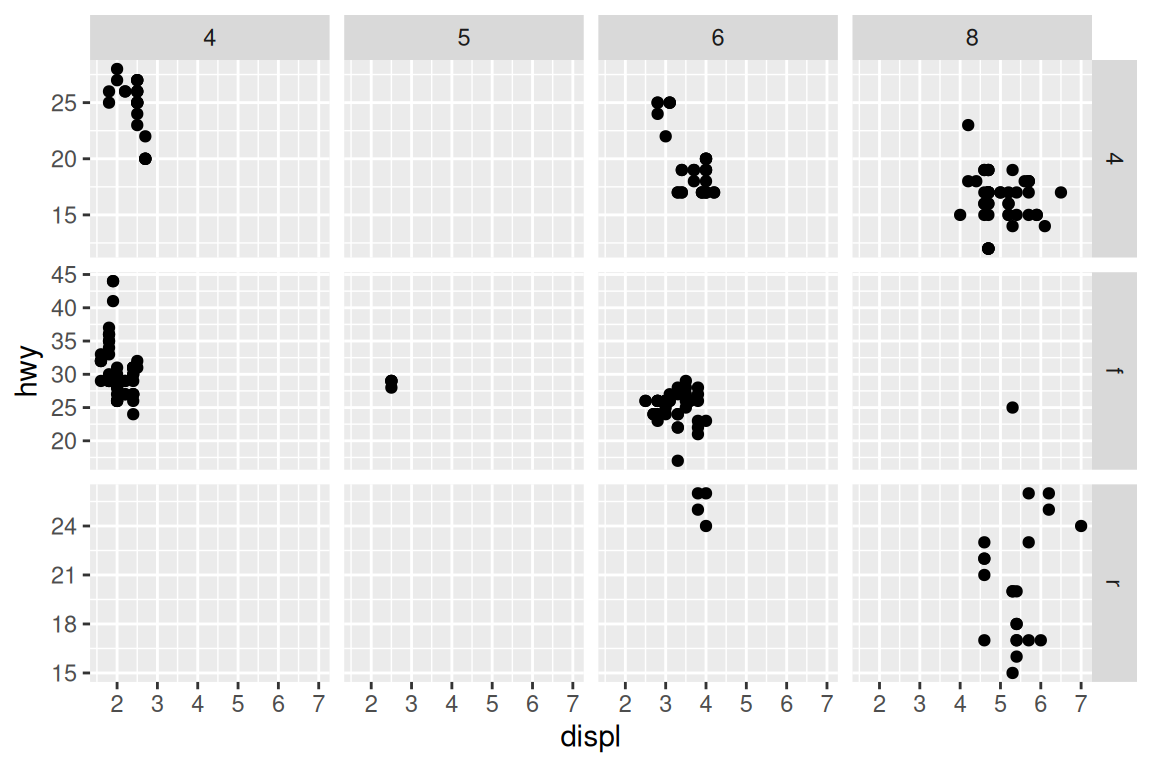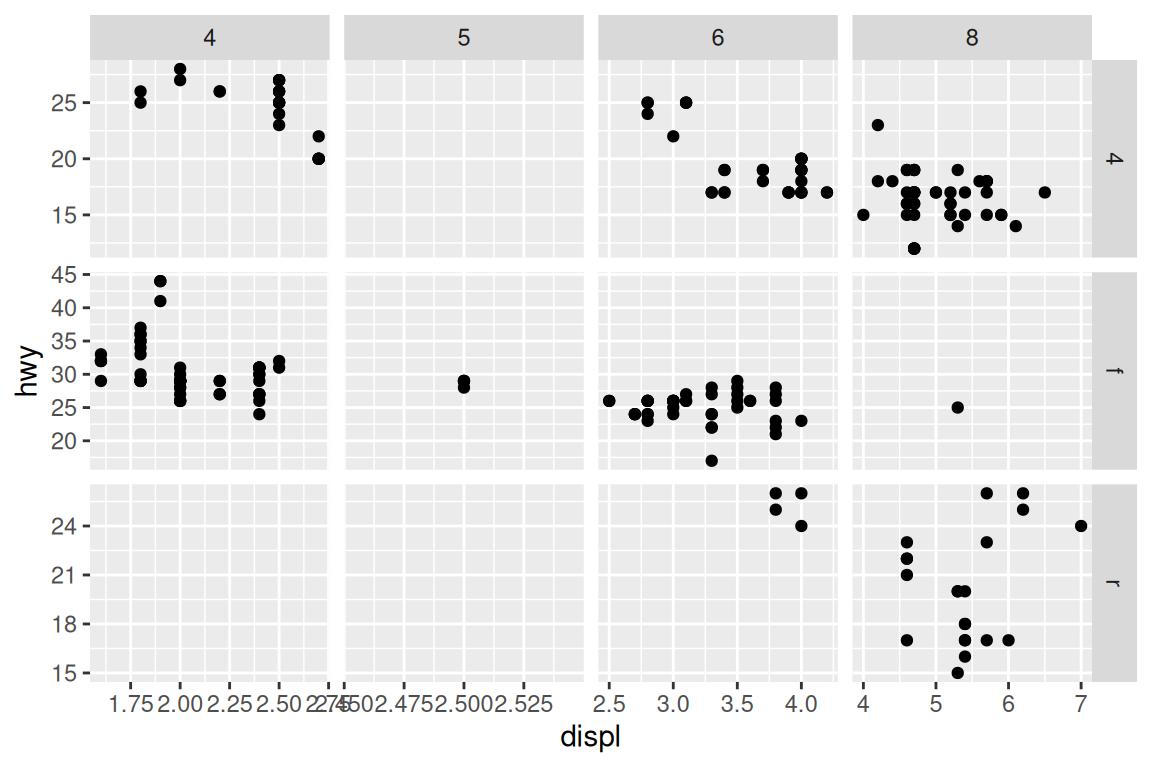Figure 11.3: With free y scales (top); With free x and y scales (bottom)

### 11.2.3 Discussion

Each row of subplots has its own y range when free y scales are used; the same applies to columns when free x scales are used.

It’s not possible to directly set the range of each row or column, but you can control the ranges by dropping unwanted data (to reduce the ranges), or by adding `geom_blank()` (to expand the ranges).

See Recipe 3.10 for an example of faceting with free scales and a discrete axis.

## 11.3 Changing the Text of Facet Labels

### 11.3.1 Problem

You want to change the text of facet labels.

### 11.3.2 Solution

Change the names of the factor levels (Figure 11.4):

``````library(dplyr)

# Make a modified copy of the original data
mpg_mod <- mpg %>%
# Rename 4 to 4wd, f to Front, r to Rear
mutate(drv = recode(drv, "4" = "4wd", "f" = "Front", "r" = "Rear"))

# Plot the new data
ggplot(mpg_mod, aes(x = displ, y = hwy)) +
geom_point() +
facet_grid(drv ~ .)``````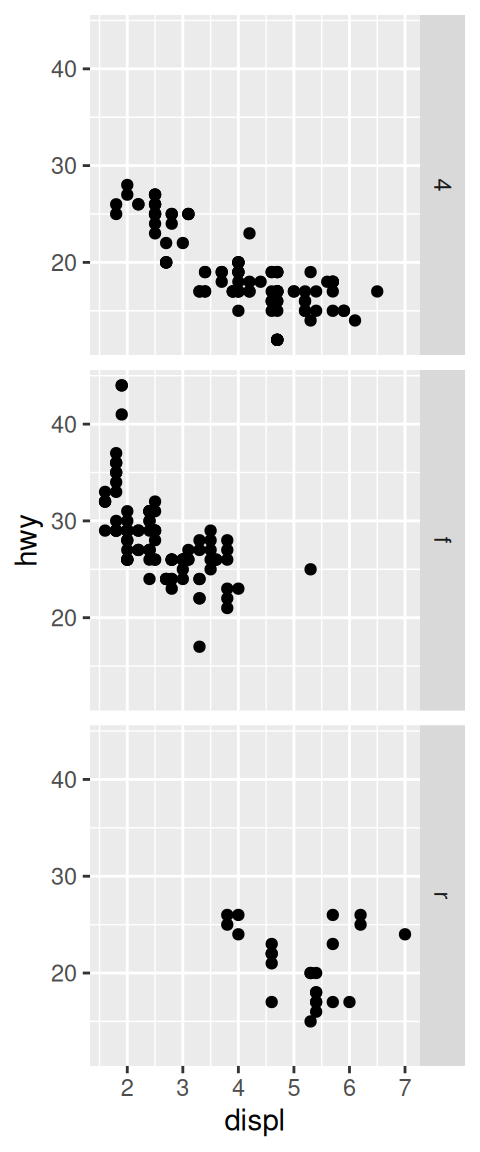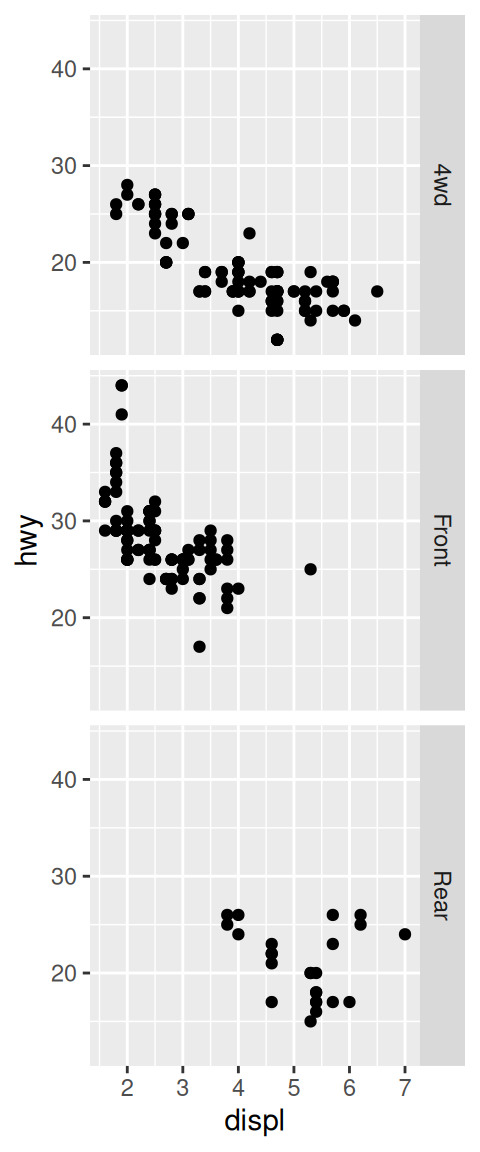Figure 11.4: Default facet labels (left); Modified facet labels (right)

### 11.3.3 Discussion

Unlike with scales where you can set the labels, to set facet labels you must change the data values. Also, at the time of this writing, there is no way to show the name of the faceting variable as a header for the facets, so it can be useful to use descriptive facet labels.

With `facet_grid()` but not `facet_wrap()`, at this time), it’s possible to use a labeller function to set the labels. The labeller function `label_both()` will print out both the name of the variable and the value of the variable in each facet (Figure 11.5, left):

``````ggplot(mpg_mod, aes(x = displ, y = hwy)) +
geom_point() +
facet_grid(drv ~ ., labeller = label_both)``````

Another useful labeller is label_parsed(), which takes strings and treats them as R math expressions (Figure 11.5, right):

``````# Make a modified copy of the original data
mpg_mod <- mpg %>%
mutate(drv = recode(drv,
"4" = "4^{wd}",
"f" = "- Front %.% e^{pi * i}",
"r" = "4^{wd} - Front"
))

ggplot(mpg_mod, aes(x = displ, y = hwy)) +
geom_point() +
facet_grid(drv ~ ., labeller = label_parsed)``````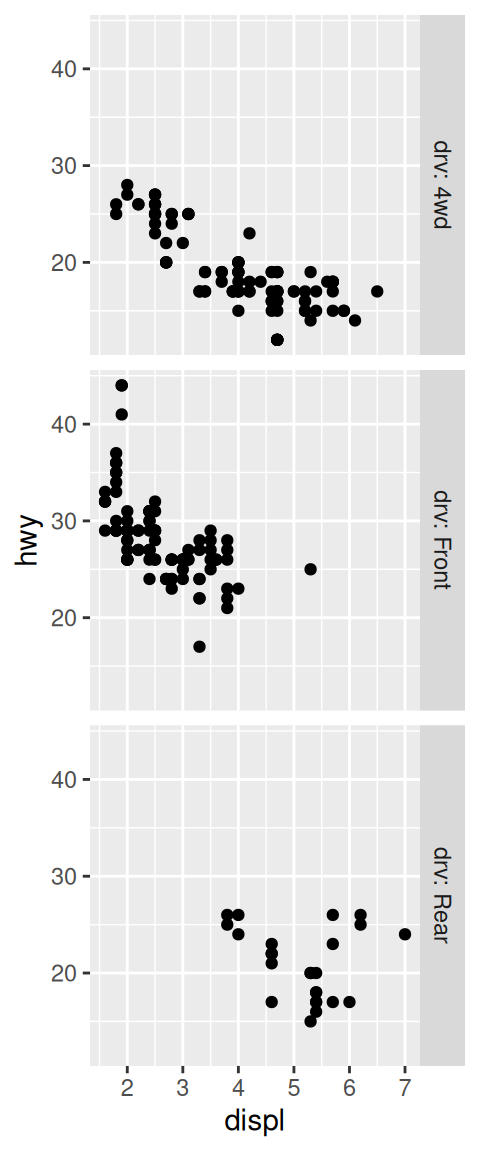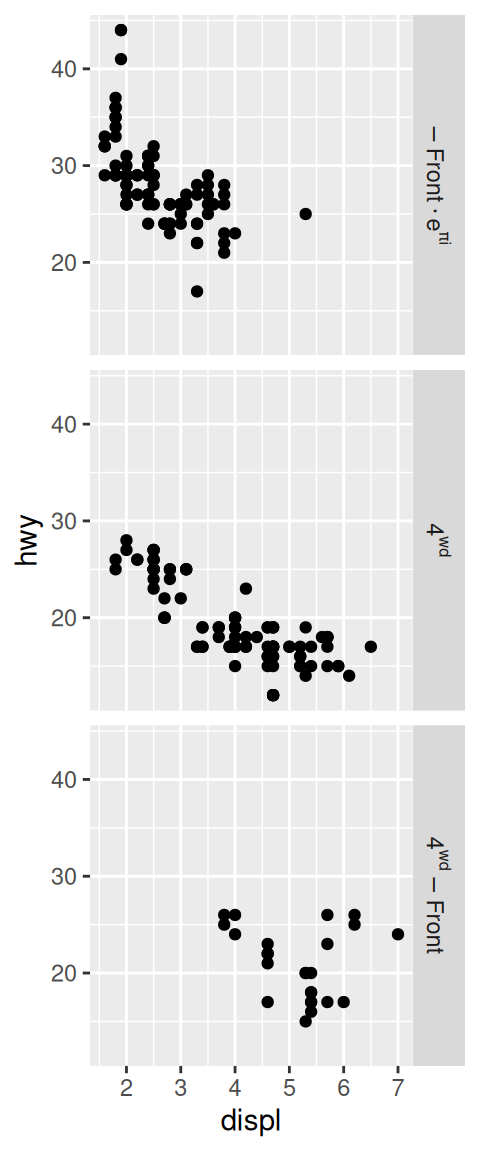Figure 11.5: With `label_both()` (left); With `label_parsed()` for mathematical expressions (right)

See Recipe 15.10 for more on renaming factor levels. If the faceting variable is not a factor but a character vector, changing the values is somewhat different. See Recipe 15.12 for information on renaming items in character vectors.

## 11.4 Changing the Appearance of Facet Labels and Headers

### 11.4.1 Problem

You want to change the appearance of facet labels and headers.

### 11.4.2 Solution

With the theming system, set `strip.text` to control the text appearance and `strip.background` to control the background appearance (Figure 11.6):

``````library(gcookbook)  # Load gcookbook for the cabbage_exp data set

ggplot(cabbage_exp, aes(x = Cultivar, y = Weight)) +
geom_col() +
facet_grid(. ~ Date) +
theme(
strip.text = element_text(face = "bold", size = rel(1.5)),
strip.background = element_rect(fill = "lightblue", colour = "black", size = 1)
)``````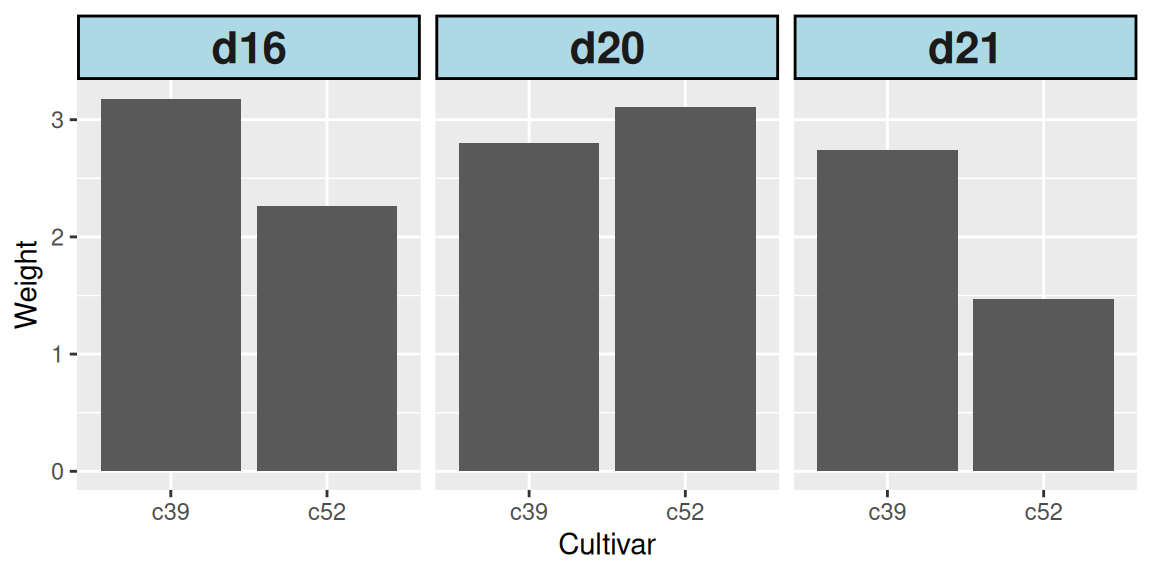Figure 11.6: Customized appearance for facet labels

### 11.4.3 Discussion

Using `rel(1.5)` makes the label text 1.5 times the size of the base text size for the theme. Using `size = 1` for the background makes the outline of the facets 1 mm thick.